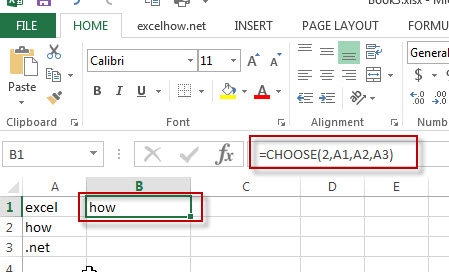# Excel Choose Function

This post will guide you how to use Excel CHOOSE function with syntax and examples in Microsoft excel.

### Description

The Excel CHOOSE function returns a value from a list of values.

The CHOOSE function is a build-in function in Microsoft Excel and it is categorized as a Lookup and Reference Function.

The CHOOSE function is available in Excel 2016, Excel 2013, Excel 2010, Excel 2007, Excel 2003, Excel XP, Excel 2000, Excel 2011 for Mac.

### Syntax

The syntax of the CHOOSE function is as below:

=CHOOSE (index_num, value1,[value2],…)

Where the CHOOSE function arguments are:
index_num -This is a required argument. Specify the position number in the list of values.
Value1,[value2] – This is a required argument.  A list of one or more values that you want to return
Note: The index_num value must be a number between 1 and 29.
If index_num value is less than 1 or greater than the length of the value list, the CHOOSE function will return #VALUE! Error value.

### Example

The below examples will show you how to use Excel CHOOSE Lookup and Reference Function to return a value from a value list based on a position value.

#1 To get the second value in the value list in B1 Cell, just using the following excel formula: =CHOOSE(2,A1,A2,A3)### More Excel Choose Function Examples

• Get the First Monday of a Given Year
To calculate the first Monday of the year or given any date, you can create a new complex formula based on the DATE function, the YEAR function and the WEEKDAY function……
• List all Worksheet Names
Assuming that you have a workbook that has hundreds of worksheets and you want to get a list of all the worksheet names in the current workbook. And the below will introduce 3 methods with you..…
• Copy and Paste Only Non-blank Cells
If you want only copy non-blank cells in a range in Excel, you need to select the non-blank cells firstly, then press Ctrl +C keys to copy the selected cells. So how to only select all non-blank cells in the selected range in your worksheet..…